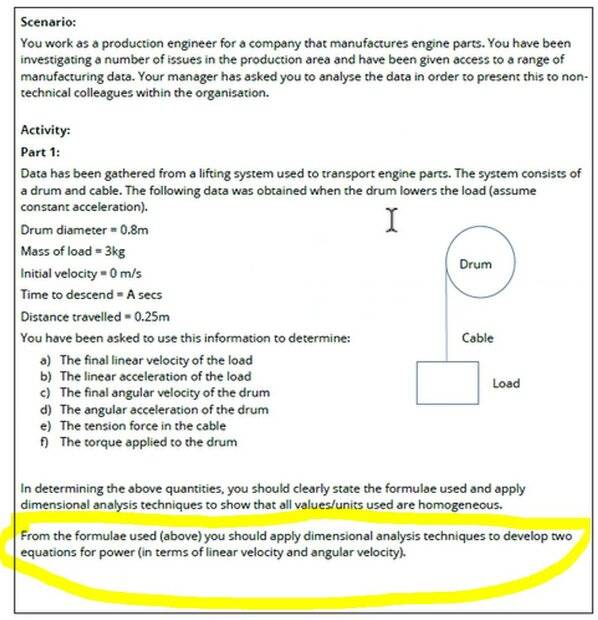# Dimensional Analysis

• etile
etile
Homework Statement
university
Relevant Equations
n/a
Hi guys,

Please see attached image - it's the part highlighted yellow that I'm stuck on.

Here is what I got for linear velocity and angular velocity. (requested by mod)

Thanks!

c) Angular to velocity
v (linear velocity) = r (radius of circle) * omega (angular velocity)
lets rearrange
linear velo (2.214 m/s = 0.4m* angular)
2.214 / 0.4

d) Angular acceleration of the drum
initial angular velo = 0
final angular veloc=5.535
time=0.225756
formula=
angular acceleration = initial angular velo. (0) - final angular acc. (5.535) / t (0.225756)Last edited by a moderator:

Staff Emeritus
Homework Helper
Gold Member
PF rules require you to provide your own efforts. What are your thoughts on the part of the problem you are asking about?

•topsquark
etile
Sorry I didn't realise that.

I pretty much don't understand the question and where to go with it

Homework Helper
Gold Member
2022 Award
•